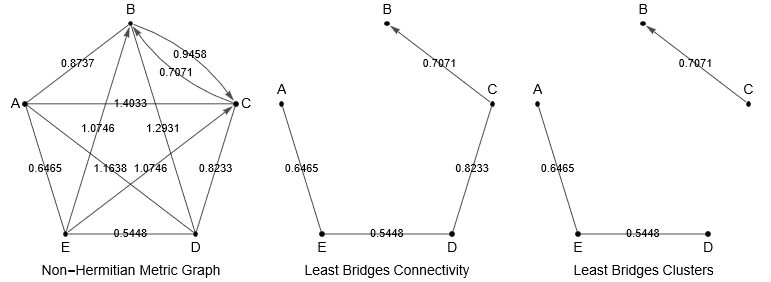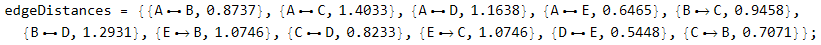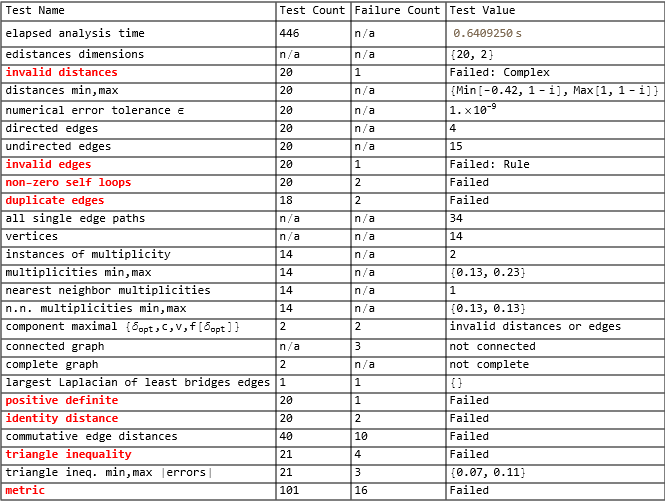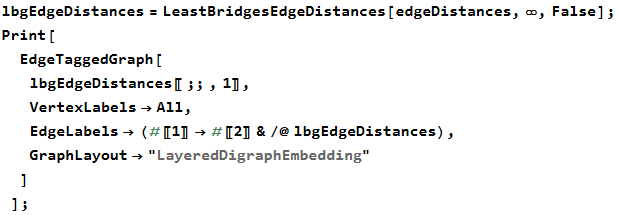Least Bridges Graphs   A computational method for analyzing distance relationships.      RRID:SCR_022115   last update 3/23/22.• Mathematica® Project FAQ
• What is it for?
• Establishing metric validity and group relationships among dissimilarity or distance measurements in abstract spaces. This toolbox was originally created for analysis of values arising in genetic distance measures. The contained algorithms correct errors in commonly used nearest-neighbor and cluster software that are not designed with distances in mind (e.g. nearest-neighbor topology functions currently found in Mathematica, Python, SPSS, etc).
• What analyses and outputs does it provide?
• The provided functions are for execution within the Mathematica notebook environment. Given a list of vertices (e.g. names of objects or specimens) and the measured dissimilarities or distances between them, the distance analysis function computes a Table of statistics and analyses of the topological system including types of edges, existence of multiple edges between two vertices, self-loops, vertices with multiplicity, maximal component distance, the maximum laplacian value of the least bridges graph, a battery of metric tests, and the metric type (if any) of metric found. Other functions in the project produce edge distance lists of clusters, core components, and nearest neighbors -- all of which can be visualized within the Mathematica environment. Several examples are provided.
• Why should I care if my distances form a metric?
• If your "distances" do not form a metric, then technically they are not distances and not valid for comparison to each other, for use in distance cluster analysis, distance dendrograms, etc.
• What is vertex multiplicity?
• The number of identical distances leading to/from a vertex. It is not an error to have them, however commonly used clustering and nearest-neighbor algorithms ignore them and consequently produce erroneous results.
• Why must a metric have no zero-length distances (positive definite)?
• It obscures topology (e.g. hides a triangle as a rectangle) from triangle inequality analysis, and thus can cause data to pass which would otherwise fail. So if you find zero distances, those vertices (or subsets of them) are identical under your dissimilarity measure. Make a note of them, and then for each set of identicals choose one to be an "ambassador" and remove its duplicates. Repeat this process for each set of identicals.
• What kind of input files can I use?
• Mathematica isn't picky. Examples are provided for CSV files containing columnar lists or matrices of dissimilarity values. The functions themselves require lists of edge distances, e.g.
•Examples of data conversion are provided.
• What kinds of distance values can I use?
• Integers, rationals, floating point, and in general: any subset of Real.
• What Mathematica version do I need?
• 12.1 or later, since the HashTable data structure is used.
• What other licenses do I need?
• This software is offered on a "use at your own risk" basis.
Permission for resale of the software in any form is not granted.
• How do I install it?
• In your "Documents" folder, create (or find) a directory named "Mathematica Projects".
• Unzip the entire file.
• Done!
• How do I check that it is installed correctly?
• See notebook 1.
• How do I read a CSV list of distance data?
• See notebook 2.
• How do I read a CSV distance matrix?
• See notebook 3.
• How do I analyze a list of distances?
• See notebook 4.
• Can you help me with my Mathematica program?
•
• Limitations: Currently, vertices must be integer, String, or Real - nothing decomposable by List@@
•
• Function Synopsis
• EdgeDistanceGraphAnalysis[edgeDistances, verbose]
• Returns resultsTable which can be rendered with
• Print[Grid[resultsTable,Alignment->Left,Frame->All]];
• When verbose is True the function prints progress messages to the notebook, which can be useful during analysis of a large number of edges.
Critical errors in the data are shown in red. For example:• Explanation of tests:
• invalid distances - must be Real or subset thereof (i.e. integer, rational, floating point).
• numerical error tolerance ϵ - computed tolerance for triangle inequality.
• invalid edges - edges may only be DirectedEdge, UndirectedEdge, or a mixture.
• non-zero self loops - an edge from a vertex to itself with non-zero distance will fail the metric identity test.
• duplicate edges - two vertices may not be connected by more than one edge in the same direction, a violation of metric tests.
• instances of multiplicity - in the context of distance, vertex multiplicity refers to multiple edges of the same distance measure originating from the same vertex (note that undirected edges have two origination vertices).
• nearest neighbor - for a given vertex, the target (incident) vertex of the least distance edge (note that nearest neighbors are not always mutual, even in the case of undirected edges).
• nearest neighbor multiplicities - the number of vertices with multiple nearest neighbors.
• component maximal - the construction of a Least Bridges Graph can be limited to a ẟ value less than the maximum nearest neighbor distance, which will result in a graph missing one or more vertices and for low enough values an increasing number of graph components. The component maximal ẟopt occurs where f[ẟ] ∝ c·v is maximized, with c = # of components and v = # vertices. All clusters in the distance graph are exhibited at this value. A telescoping search is performed to find ẟopt which could be inaccurate for unusual graphs.
• largest Laplacian of least bridges edges - an upper bound on the number of edge frequencies in the least bridges graph of all edge distances, and hence the variety of clusters within the graph.
• positive definite - all distances are greater than zero. 1st of 4 metric tests.
• identity distance - any self-loops have distance zero. 2nd of 4 metric tests. Note with positive definite this forbids self-loops.
• commutative edge distances - in the case where two vertices are mutually connected by directed edges, are both distances equal? This will determine what kind of metric is present, if any. 3rd of 4 metric tests.
• triangle inequality test - for all vertices {a,b,c} with single edge paths a to b, b to c, and a to c: the condition ẟ[a,c] ≤ ẟ[a,b] + ẟ[b,c] must hold. Note that this is what we expect from any triangle, the sum of two edge lengths cannot be greater than the third. 4th of 4 metric tests.
• Example notebooks: 4, 40, 401, 402.
•
• LeastBridgesEdgeDistances[edgeDistances, distancelimit, verbose]
• Returns lbgEdgeDistances whose contents can be rendered with Mathematica function EdgeTaggedGraph[]
•For the distancelimit L:
• L ≤ 0 → no graph computed
• L < ∞ → LBG computation halts at L if reached
• L = ∞ → no distance limit
• NumberQ[L] == False → ignored
When verbose is True the function prints progress messages to the notebook, which can be useful during analysis of a large number of edges.
• Algorithm:
• Least Bridges Graphs are a method of visualizing nearest-neighbor relationships in abstract spaces. They are constructed by first considering the vertices (e.g. genetic profiles) as disconnected components, then incrementally adding the shortest available edge connection (i.e. edge representing distance between the two components). Edges are only added between disconnected components and thus termed "bridges". A new component is created each time an edge is added, replacing the prior two. If there are multiple edges of the same distance that qualify then the entire set is added, possibly engulfing multiple components. This latter requirement ensures edge multiplicity is not ignored – an error in many cluster algorithms used for distances. The distances among components must be re-evaluated after an edge or edge set is added. Inter-component distances are determined by selecting the shortest vertex-to-vertex distance between them. The process is continued recursively until a prescribed limit is reached (e.g. a maximum distance), a connected graph is achieved, or the vertex list is exhausted.
• Example notebooks: 4, 50, 501, 502.
•
• LeastBridgesClusters[edgeDistances]
• Determines component maximal distance and returns edge distances of components found at that value.
• Input: edgeDistances, as above.
• Output: selectedEdgeDistances.
• Example notebooks: 4, 40, 402.
•
• LeastBridgesClassifiedEdges[edgeDistances, distancelimit, verbose]
• Inputs: same as LeastBridgesEdgeDistances.
• Output: {componentCoreEdges, nnBridges, leastBridges} as edge distance lists.
• componentCoreEdges → edges in new components encountered by least bridges graph algorithm
• nnBridges → bridges that are also nearest neighbors
• leastBridges → bridges that are not nearest neighbors but nonetheless the shortest available edge
• Example notebooks: 60, 601, 602, 603.
•
• LeastBridgesPartitionedEdges[edgeDistances, edgeStyle, labeled, distancelimit, verbose]
• Partitions edges according to distribution(s) found in distance histogram.
• Inputs:
• edgeDistances, as above
• edgeStyle → "plain edges" | "hued edges"
• labeled → False | True | fpp (False → no label, True → labeled with default fpp, fpp → use precision fpp >= 1)
• distancelimit, as above
• verbose, as above
• Output: {hues, partitions, graphEdgeDistances}.
• graphEdgeDistances → Annotated edges (wrapped in Annotation[]).
• partitions → list of distance partition boundaries. "plain edges" will be limited to distances min and max.
• hues → list of distance partition hues. "plain edges" will be black.
Use HuePartitionLegend[] to create a legend from hues and partitions.
• Example notebooks: 70, 700.
•
• NearestNeighborEdgeDistances[edgeDistances]
• Outputs nearest-neighbor EdgeDistances, where {Edge[a, b], c} means b is a nearest-neighbor of a at distance c.
• Input: edgeDistances, as above.
• Output: selectedEdgeDistances.
• Example notebooks: 80, 800.
•
• ComponentMaximal[edgeDistances]
• Determines the component maximal in the least bridges graph of edgeDistances.
• Input: edgeDistances, as above.
• Output: {fmaxdata, fvalsdata, testCount}.
• fmaxdata → {ẟopt, c, v, f[ẟopt]}.
• ẟopt → component maximal distance.
• c → number of components in least bridges graph at ẟopt.
• v → number of vertices in least bridges graph at ẟopt.
• f[ẟopt] → the function value maximized at ẟopt. Note that f[ẟ] ∝ c·v.
• fvalsdata → {{ẟ1, c, v, f[ẟ1]}, ..., {ẟn, c, v, f[ẟn]}} where n is determined by a telescoping algorithm.
• testCount → number of telescoping iterations performed.
• Example notebook: 900.
•
• LaplacianMatrixEigenvalues[edgeList]
• The Laplacian matrix is a divergence operator formed by subtracting the vertex adjacency matrix from the diagonal matrix of vertex degrees: L = D – A. The eigenvalues are always decreasing in magnitude and end in zero. The first and largest of them λmax is an upper bound on all edge frequencies and hence the variety of substructures within the graph.
• Execution scales in polynomial time to the graph complexity. Ill-conditioned adjacency matrices will generate errors.
• Input: A list of edges, e.g. edgeDistances[[;;,1]]
• Output: List of Laplacian matrix eigenvalues or List of error code(s).
• Example notebook: 900.